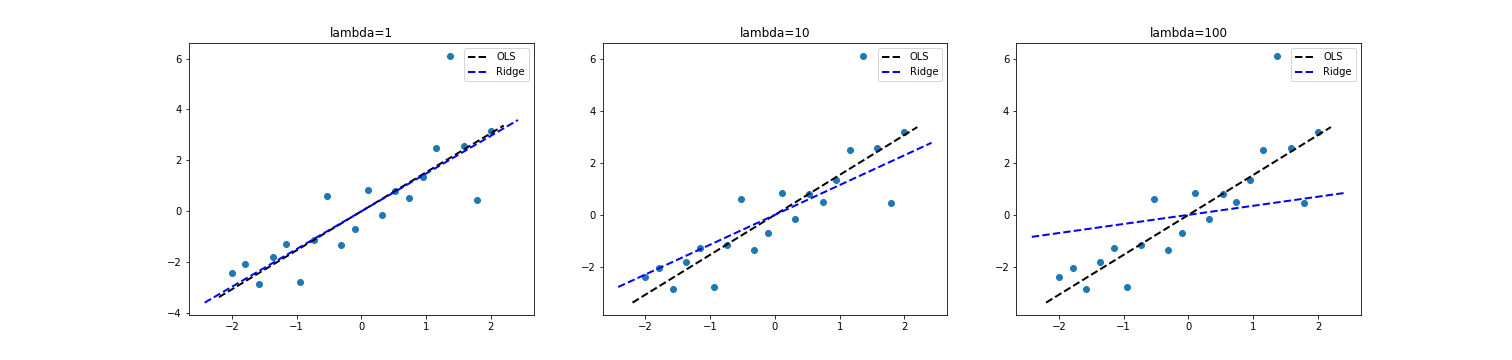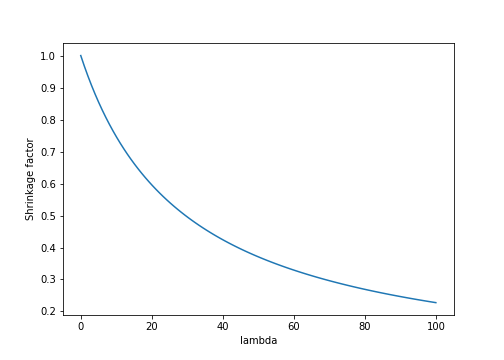# Shrinkage in ridge regression

A brief review of shrinkage in ridge regression and a comparison to OLS.

## OLS review

Consider the regression problem

$Y = X\beta + \epsilon$

where $Y$ is an $n$-vector of responses, $X$ is an $n \times p$ matrix of covariates, $\beta$ is a $p$-vector of unknown coefficients, and $\epsilon$ is i.i.d. noise. We can estimate $\beta$ by minimizing the sum of squares:

$\ell = \frac12 \|Y - X\beta\|^2_2.$

Taking the derivative w.r.t. $\beta$, we have

$\frac{\partial \ell}{\partial \beta} = -X^\top (Y - X\beta).$

Setting to zero, we have $X^\top Y = X^\top X\beta$, which implies

$\widehat{\beta} = (X^\top X)^{-1} X^\top Y.$

## MLE interpretation of OLS

Note that an equivalent solution can be found by maximizing the likelihood of the model

$Y \sim \mathcal{N}(X\beta, \sigma^2 I_p).$

Consider maximizing the log likelihood:

$\text{arg}\max_\beta \log p(Y | X, \beta) = \text{arg}\max_\beta -\frac{p}{2} \log 2\pi -\frac{p}{2} \log \sigma^2 - \frac{1}{2\sigma^2} (Y - X\beta)^\top (Y - X\beta).$

Ignoring constant terms, we have

$\frac12 p \log \sigma^2 = \frac{1}{2\sigma^2} (Y^\top Y - Y^\top X\beta - \beta^\top X^\top Y + \beta^\top X^\top X\beta)$

Taking a derivative w.r.t. $\beta$, we have

\begin{align} &\frac{\partial \ell}{\partial \beta} = 0 = \frac{1}{2\sigma^2} (- X^\top Y - X^\top Y + 2 X^\top X \beta) \\ \implies& X^\top X \beta = X^\top Y \\ \implies& \widehat{\beta}_{\text{MLE}} = (X^\top X)^{-1} X^\top Y \end{align}

## Ridge regression

Consider instead maximizing the sum of squares with an additional $\ell_2$ penalty on $\beta$:

$\min_\beta \frac12 \|Y - X\beta\|^2_2 + \frac{\lambda}{2} \|\beta\|_2^2.$

Taking a derivative w.r.t. $\beta$,

$-X^\top (Y - X\beta) + \lambda \beta = 0.$

This implies that $X^\top X\beta + \lambda \beta = X^\top Y$, and thus $(X^\top X + \lambda I_p) \beta = X^\top Y$. The solution can easily be seen as

$\widehat{\beta}_{\text{ridge}} = (X^\top X + \lambda I_p)^{-1} X^\top Y.$

In other words, we add a small constant value $\lambda$ to the diagonal of the sample covariance $X^\top X$ before inverting it.

To see this another way, consider the SVD of $X$,

$X = UDV^\top.$

Plugging this into the ridge regression solution, we have

\begin{align} \widehat{\beta} &= (VDU^\top UDV^\top + \lambda I_p)^{-1} VDU^\top Y \\ &= (VD^2V^\top + \lambda I_p)^{-1} VDU^\top Y \\ &= (D^2 + \lambda I_p)^{-1} VDU^\top Y. \end{align}

The fitted values $X \widehat{\beta}$ are then

\begin{align} X \widehat{\beta} &= X (D^2 + \lambda I_p)^{-1} VDU^\top Y \\ &= UDV^\top (D^2 + \lambda I_p)^{-1} VDU^\top Y \\ &= UD (D^2 + \lambda I_p)^{-1} DU^\top Y \\ &= \sum\limits_{j=1}^p u_j \frac{d_j^2}{d_j^2 + \lambda} u_j^\top Y \end{align}

The “shrinkage factor” for feature $j$ is $\frac{d_j^2}{d_j^2 + \lambda}$. For mean-centered data, this essentially corresponds to a rotation of the coefficient vector toward zero. In effect, this means that the fitted values $\widehat{Y}$ are shrunk toward zero. Notice that if $\lambda=0$, it reduces to vanilla least squares with $X \widehat{\beta} = UU^\top Y$. This is the projection of Y onto the orthogonal subspace defined by $X$.

We can visualize this. Below is a scatter plot with $p=1$ and $n=20$ points where the true value $\beta=1.5$. As we plot the OLS and ridge estimates, we can see that the ridge coefficient rotates toward zero as $\lambda$ increases.Furthermore, we can see that the shrinkage factor $\frac{d^2}{d^2 + \lambda}$ will decrease (causing more shrinkage) at the rate $\frac{c}{c + \lambda}$, where $c$ is a constant determined by the $j$th singular value of $X^\top X$:In higher dimensions, each covariate will have a different rate of shrinkage (determined by its corresponding singular value).

## MLE interpretation of ridge regression

Notice that we can arrive at an equivalent solution to ridge regression assuming the following model, where we place a prior on $\beta$

$Y \sim \mathcal{N}(X \beta, \sigma^2 I), ~~~ \beta \sim \mathcal{N}(0, \frac{1}{\lambda} I).$

The posterior is

$p(\beta | X, Y) = \frac1Z p(Y | X, \beta) p(\beta)$

where $Z$ is the normalizing constant. We can find a MAP solution by maximizing the quantity without the constant, which is proportional to the posterior.

\begin{align} &\max_\beta \log p(Y | X, \beta) + \log p(\beta) \\ &= \max_\beta -\frac{p}{2} \log 2\pi -\frac12 p \log \sigma^2 - \frac{1}{2\sigma^2} (Y - X\beta)^\top (Y - X\beta) - \frac{p}{2} \log 2\pi -\frac{p}{2} \log \sigma^2 - \frac{\lambda}{2} |\beta|_2^2. \end{align}

Ignoring constant terms and taking a derivative w.r.t. $\beta$, we have

\begin{align} &-\frac{1}{2\sigma^2} \left( -X^\top Y - X^\top Y + 2 X^\top X \beta\right) -\frac{\lambda}{2} 2\beta = 0 \\ \implies& X^\top X \beta + \sigma^2 \lambda \beta = X^\top Y \\ \implies& \widehat{\beta} = \left( X^\top X + \sigma^2 \lambda I\right)^{-1} X^\top Y \end{align}

## The ridge solution to collinearity

Suppose our data lives in $\mathbb{R}^2$, that is, $X \in \mathbb{R}^{n \times 2}$. Further, suppose the two columns of $X$ are identical. If we then perform linear regression with response $Y$, the problem is under-constrained: there are an infinite number of equally good solutions. To see this, consider an SVD of $X = UDV^\top$, and notice that

$(X^\top X)^{-1} X^\top Y = UU^\top Y = \sum\limits_{j=1}^p u_j u_j^\top Y = u_1 u_1^\top Y + u_2 u_2^\top Y.$

Since the columns of $X$ are equal, we know that $u_1 = u_2$. In this case, we can arbitrarily scale each term to get an infinite number of equivalent solutions:

$\widehat{\beta} = \gamma u_1 u_1^\top Y + \frac{1}{\gamma} u_2 u_2^\top Y, ~~ \forall \gamma\in \mathbb{R}.$

Ridge regression alleviates this issue by adding a small quantity to the diagonal of $X^\top X$ to make the solution unique:

$u_1 \frac{d_1^2}{d_1^2 + \lambda} u_1^\top Y + u_2 \frac{d_2^2}{d_2^2 + \lambda} u_2^\top Y.$